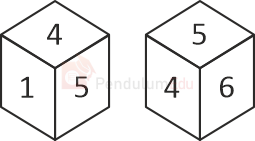Quantitative Aptitude or Quant comprises the topics from Mathematics, which are used to assess the problem-solving ability and intelligent quotient of any student. Generally, students are afraid of this section while preparing for competitive exams. Are you one of them? Even if you are, then you don’t have to worry about this section as PendulumEdu has come up with the best study material for every topic which is asked in any competitive exam. Experienced faculties and exam experts prepare the study material. In our study material, you will get all the theory, basic formulae, quick approaches, and tricks, which are essential to crack any exam. Entire study material is complete, sufficient and prepared with meticulous research on exam patterns and level of various exams like SSC exams, Railways Exams, BANKING exams, UPSC exams, STATE PCS exams and various other regional exams to boost your scores in any government exam even if you don’t have in-depth knowledge of a topic.

Quant section can broadly be classified into:

1. Arithmetic
3. DATA INTERPRETATION

The arithmetic section involves topics like Percentage, Profit and loss, Simple interest and compound interest, Ratio and Proportion, Time and Work, Time, speed and distance, Averages, Mixture and Allegation, Number System, etc.

While Advanced maths section involves topics like Algebra, Trigonometry, Geometry, Coordinate Geometry, Mensuration, Permutation and combination, probability, etc.

DATA INTERPRETATION: Data interpretation involves analyses and inspection of data in the form of tables, bar charts, line charts, caselet, and various other types to interpret various results based on the data. This section also involves topics like Mean, Median, Mode, Variance, Standard deviation, correlation and regression.

So, prepare your topics with the PendulumEdu and get your dream JOB. Use the Quant Study Material by PendulumEdu and clear your concepts, learn quick approaches, and score 100% marks in the exams.

Arithmetic

Geometry

Read about the basics and tricks to apply to the questions related to the lines and angles, triangles, circles, coordinate geometry, etc. in the exam sections like SSC CGL Quant, IBPS PO/CLERK/SO/RRB Quant, UPSC CDS maths, SBI PO/CLERK/SO Quant and any other exam where geometry is part of the syllabus

Current Affairs

Attempt Quiz

## Question Of The Day

Two different positions of the same dice are shown. Which number will be at the top if ‘1’ is at the bottom?